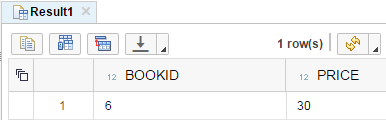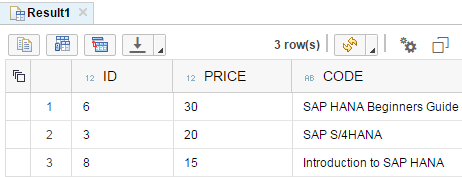﻿ SQLScript Nth_Value Function in SAP HANASAP ABAP Programming and HANA Database Tutorials Development resources, articles, tutorials, code samples, tools and downloads for SAP HANA and ABAP, HANA Database, SQLScript, SAP UI5, Screen Personas, Web Dynpro, Workflow

# SQLScript Nth_Value Aggregation Function in SAP HANA

Using SQLScript Nth_Value() function, SQL developers can return the value of an element which is ordered by a specified column ascending or descending order and has a certain position in the ordered list.

For example, using NTH_VALUE function SQLScript developer can return the first row in the SAP HANA database table.

An other example can be to return the top 5 rows (where NTH_VALUE is 5 or less) when SAP HANA database table data is ordered according to a rule specified in the function arguments.

Here is the SQLScript syntax for NTH_VALUE() function for developers.

Above Nth_Value() function enables SQLScript developers to return the field value of column1 at position n when data is ordered by column2.

Let's make an example.
Assume we have a SAP HANA database table named Books where we store book attributes like name and price.
In below SQLScript, you will find table creation and population with sample data for this tutorial.

Let's now select the book which is the first most expensive book when all books are ordered according their price.

The output of above SQLScript Select statement where Nth_Value() function is used for first row is as follows returning the book with price 30And what about for the first 3 rows for example.
Or in our tutorial sample, which books are the top three expensive books stored in SAP HANA database table Books?
Here comes the answer with a SQLScript Select statement.

As you see, the top 3 books are filtered by price column for values equal to or greater than the 3rd expensive book's price.Of course, SQLScript developers are free to use more than one column in the ORDER BY list.

This sample is interesting because I'm trying to find the book which is least expensive from the cheapest book :)
In short, the second cheapest book can be found by executing the following SQL Select statement.

Although there are two books with the same price, 10 and which are the second cheapest books of the HANA database table, only one of them is returned by the NTH_VALUE SELECT query.

So it is better to keep in mind, SQLScript NTH_VALUE function returns only a single value even there are more than one column1 values for targeted column2 in the syntax.

SAP HANA and ABAP